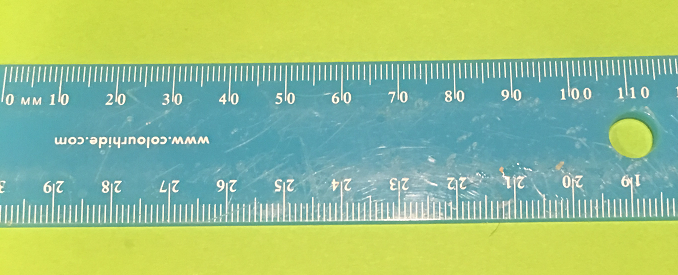# Definition of MillimetreA millimetre is a metric unit of distance that is used to measure objects with small lengths.

Carpenters often take measurements in millimetres.

One metre contains $1,000$ millimetres.

The abbreviation of millimetre is mm

For example,

• There are $10$ mm in one centimetre.
• A washer is usually $1$-$2$ mm thick.
• The ruler in the picture shows measurements in millimitres.

### Description

The aim of this dictionary is to provide definitions to common mathematical terms. Students learn a new math skill every week at school, sometimes just before they start a new skill, if they want to look at what a specific term means, this is where this dictionary will become handy and a go-to guide for a student.

### Audience

Year 1 to Year 12 students

### Learning Objectives

Learn common math terms starting with letter M

Author: Subject Coach
You must be logged in as Student to ask a Question.